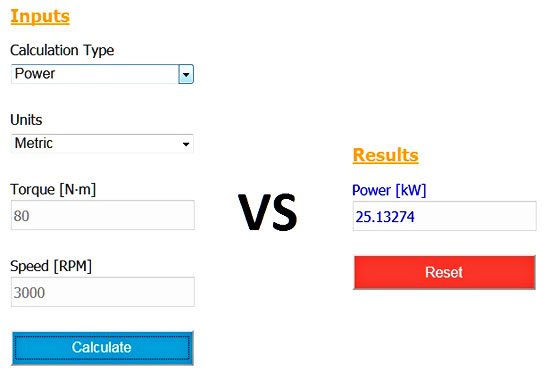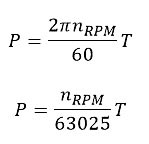# Power – Torque Online Calculator

January 28, 2019### Introduction

A year ago, I created my first online calculation. I think that now is the right time to publish a second one. This calculator was developed due to a personal need. At some point during the previous weeks, I had to calculate the power from an engine, given its rotating speed and torque. So, after performing the calculation in Excel, I decided to create an online version of it.

This tool calculates the power, given the torque and vice versa. In both cases, the rotating speed is an additional input. The formulas used in the calculations are given below. You can read more about these formulas here.Where:
P: power [kW or HP]
T: torque [N∙m or lb∙in]
n: rotating speed [RPM]
The first formula is for metric units, whereas the second for imperial ones.

The calculator’s usage is quite straightforward: just select the calculation type (power or torque), your unit system (metric or imperial), fill in the input values, and press the Calculate button. If you want to re-use it, just clean the inputs and the result by pressing the Reset button.

### The power – torque calculator

#### Results

I hope that it will be useful for your calculations!

Category: Freeware Tools